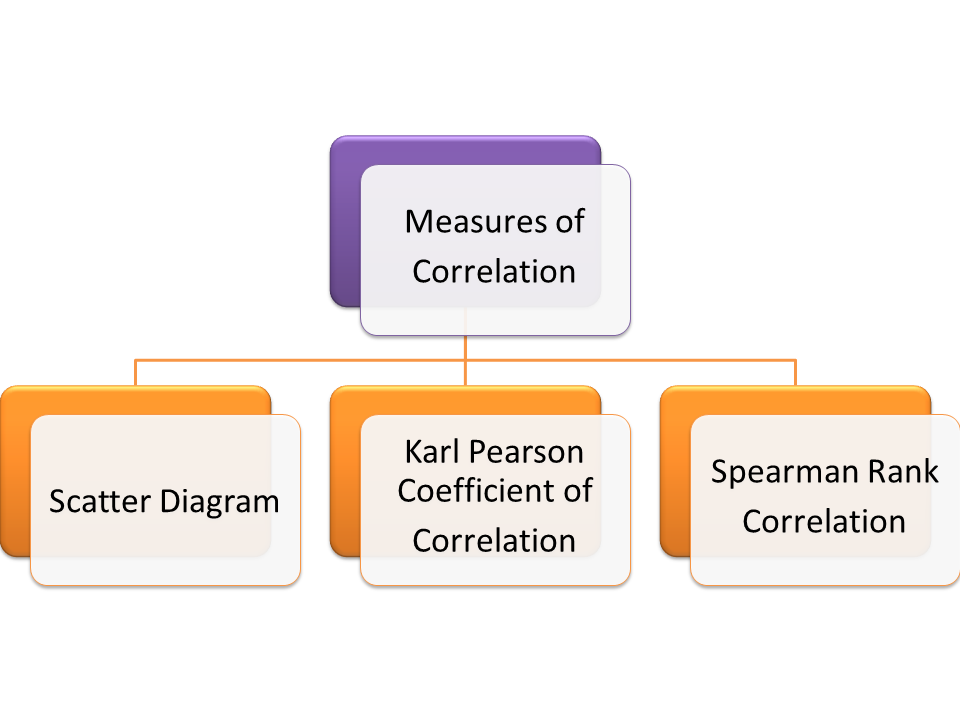## Measures of CorrelationMeasures of Correlation
There are several method of measuring correlation between two or more variables. Some of them are mention here under.

### Scatter Diagram

Scatter diagram is most popular and easy way of deciding the relation between two variables. It is a graphical method and ascertain the direction of correlation between two variables. To construct the scatter diagram take independent variable on X-Axis and dependent variable on Y Axis. Plot the graph of intersection points of two variables and decide the relation according to the scatter plot.
1. If all the points fall on a straight line moving from left lower corner to right upper corner then it is the perfect positive correlation.
2. If all the points fall on a straight line moving from upper left corner to right lower corner then it is a perfect negative correlation.
3. If all the points scattered nearby around the line then this will be high or low degree of positive and negative correlation according to the direction of line.
4. If all the points scattered every where on the graph and no pattern will be identified then there is no correlation between the variables.

### Karl Pearson's Coefficient of correlation

Scatter diagram is a graphical method of finding the correlation between two variables but it does not give the algebraic value of correlation between two variables. This become the drawback of the scatter diagram method. To overcome this Karl Pearson suggested a quantitative method of measurement of correlation which is known as the Karl Pearson coefficient of correlation denoted by (r). This gives the degree of correlation between the variables. Karl Pearson suggested the following methods to measure the coefficient of correlation.

#### Deviation Method

In this method first the deviation of each variable is find out from its mean value i.e. if X and Y are two variable then the deviations are (X-Xbar) and (Y-Ybar). Now find the sum of the multiplication of the deviation. Then divide this sum by square root of sum of the square of the deviation of each variable.

#### Product Moment Method

Different from deviation method the product moment method is treated the actual values of the variables multiplying the sum of the product of individual value of the variable by the number of variables and subtracting product of sum of individual values of variables. Divide this value by {square root (N. sum of square of X-square of sum of X). square root(N.sum of square of Y-square of sum of Y)}

#### Variance-Covariance method

In this method to find the coefficient of correlation formula of covariance and variance is used.

### Spearman's Rank Correlation

Karl Pearson coefficient of correlation deal with quantitative data. But it doesn't suggest the method of treating the qualitative data. Here Spearman's Rank correlation works. This method used to find the coefficient of correlation when qualitative data is given like beauty, intelligence, sincerity, etc. There are three types of cases in Spearman's Rank Correlation method

#### When Ranks are given

In this case ranks have given for the qualitative data. To get the coefficient of correlation in this method we need to find the difference between the ranks then squared. Now find the sum of this squared quantities.
The formula used is
R=1-{6. Sum of D^2/(N^3-N)}
Where 'D' is difference of Ranks

#### When Ranks are not given

In this case, we need to assign the ranks. Rank first is given to highest one and so on. After ranking, proceed as in the case of given ranks method.

#### When equal of tied ranks

In this case calculate the average of same rank and assign this average rank to all the equal cases. Calculate a correction factor which will added in the formula as many times as the number of equal cases.